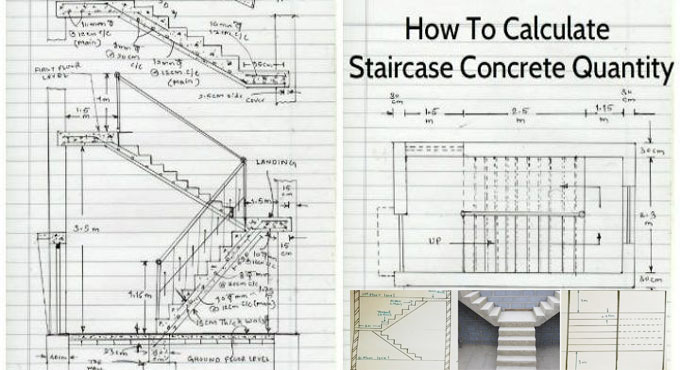# Calculation Method of Concrete Quantity for a StaircaseIn order to work out the quantity of a staircase, it is required to determine the concrete volume for the followings :-

? Steps and waist slab of 1st flight
? Steps and waist slab of 2nd flight, and
? Landing

Concrete volume for 1st flight of the staircase: The calculation is done based on the following dimensions :-

Riser = 6 inches
Length of a step = 4 feet
Number of steps = 9
The thickness of the waist slab = 6 inches

Concrete volume for the waist slab: To get the concrete volume for the waist slab, the inclined length of the waist slab be measured first. Inclined length of waist slab can be measured from the architectural plan.

The horizontal length of a waist slab is determined by,
= 9 x 10?
= 7'-6?

The height of the landing top from floor is,
= number of riser x height of riser
= 10 x 6" [number of riser = number of steps = 1]
= 5 feet

Therefore, the inclined length of the waist slab should be as follow :-
=v{(horizontal length)2 + (Height)2}
= (7?-6?)2 + (5?)2
= 9? (round up)

Therefore, the concrete volume for the waist slab should be calculated with the following formula :-
= inclined length of waist slab x width of waist slab (width of a step) x thickness of waist slab
= 9? x 4? x 6?
= 18 cubic feet

Concrete volume for steps: Since steps come as triangular shape, so the volume of a step should be as follow :-
= ? x tread x riser x length of a step
= ? x 10? x 6? x 4
= 0.84 cubic feet

As there are 9 numbers of steps in a flight, therefore, the concrete volume for the steps of the first flight should be as follow :-
= 9 x 0.84
= 7.56 cubic feet

Therefore, the entire concrete volume for the 1st flight of the staircase should be computed as follow :-
= Waist slab concrete + steps concrete
= 18 + 7.56
= 25.56 cubic feet

Concrete volume for 2nd flight of the staircase: In case the 1st flight and 2nd flight are equivalent in drawing, the concrete volume will be similar. That is,
= 25.56 cubic feet

Concrete volume for the stair landing: The calculation is done on the basis of the following dimension :-
Length of landing = 8?-6?
Width of landing = 4?-6?
The thickness of landing = 6?

Therefore, the concrete volume for the landing will be as follow :-
= 8?-6? x 4?-6? x 6?
= 19.12 cubic feet

Therefore, the total concrete volume for the staircase should be as follow :-
= 1st flight concrete + 2nd flight concrete + landing concrete
= 25.56 + 25.56 + 19.12
= 70.24 cubic feet